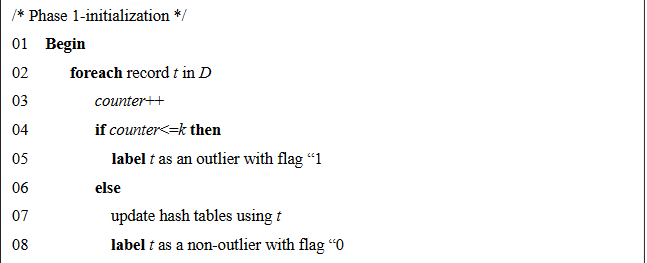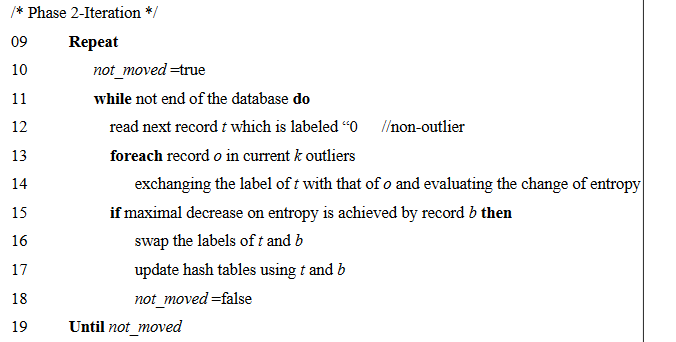### Research Paper Summary - Detecting Outliers in Categorical Data

Original Paper: An Optimization Model for Outlier Detection in Categorical Data
Authors : Zengyou He, Xiaofei Xu, Shengchun Deng

### What are outliers?

An out-lier is an observation that lies at an abnormal distance from other values in a random sample from a population. Before abnormal observations can be singled out, it is necessary to characterize normal observations.
There are many ways to detect outliers in continuous variables but there exist only few techniques which can detect outliers in categorical variables.

Example:
Suppose you have 1000 people choose between apples and oranges. If 999 choose oranges and only one person chooses apple, I would say that that person is an out-lier.
We use measurement as a way to detect anomalies. With categorical data you have to explain why choosing an apple is considered an anomaly (that data point does not behave as the rest 99.9% of the population).
One technique to detect outliers in categorical variables is using an optimization model called Local Search Algorithm which is based on expectation-maximization algorithm.

### Local Search Algorithm

The Local Search Algorithm(LSA) takes number of desired outlier's k as an input and iteratively improves the value of objective function. The objective function here is the decrease in entropy after removing the total 'k' number of outliers. The algorithm proceeds in two phases : Initialization(Phase-1) and Iteration(Phase-2).
Initialization (Phase-1):
Initially, we randomly select k points and label them as outliers. The Expectation (E) step here is to calculate the decrease in entropy after removing the points labeled as outliers.Iteration (Phase-2):
In the iteration phase, i.e. maximization (M) step, for each point labeled as non-out-lier, its label is exchanged with each of the k outliers and the entropy objective is re-evaluated. If the entropy decreases, the point’s non out-lier label is exchanged with the out-lier label of the point that achieved the new best value and the algorithm proceeds to the next object. When all non-out-lier points have been checked for possible improvements, a sweep is completed. If at least one label is exchanged in the sweep, we initiate a new sweep. The algorithm terminates when a full sweep does not change any labels, thereby indicating that a local optimum is reached.

###Reference:
1. https://arxiv.org/ftp/cs/papers/0503/0503081.pdf
2. https://math.stackexchange.com/questions/219264/are-outliers-possible-with-categorical-data